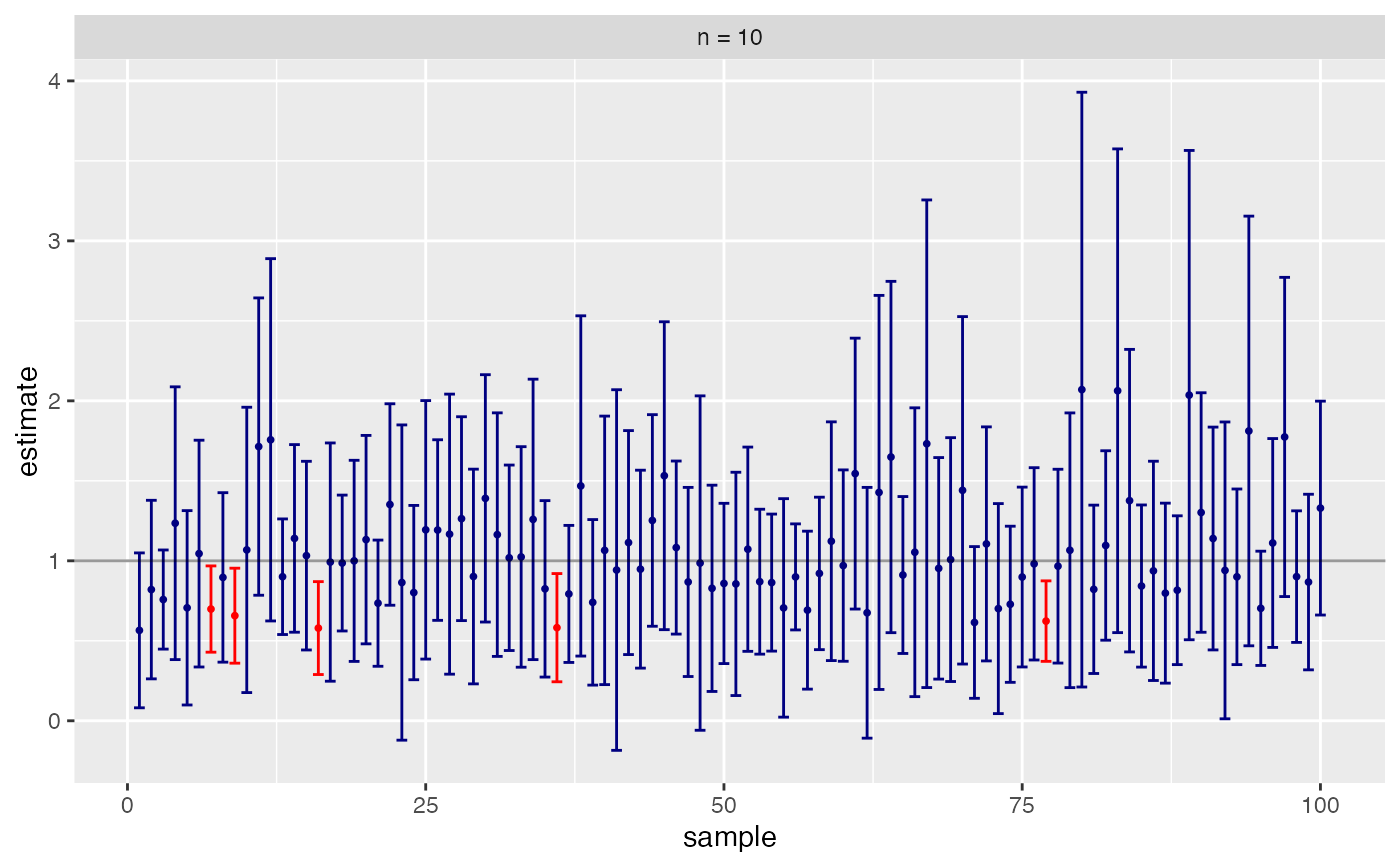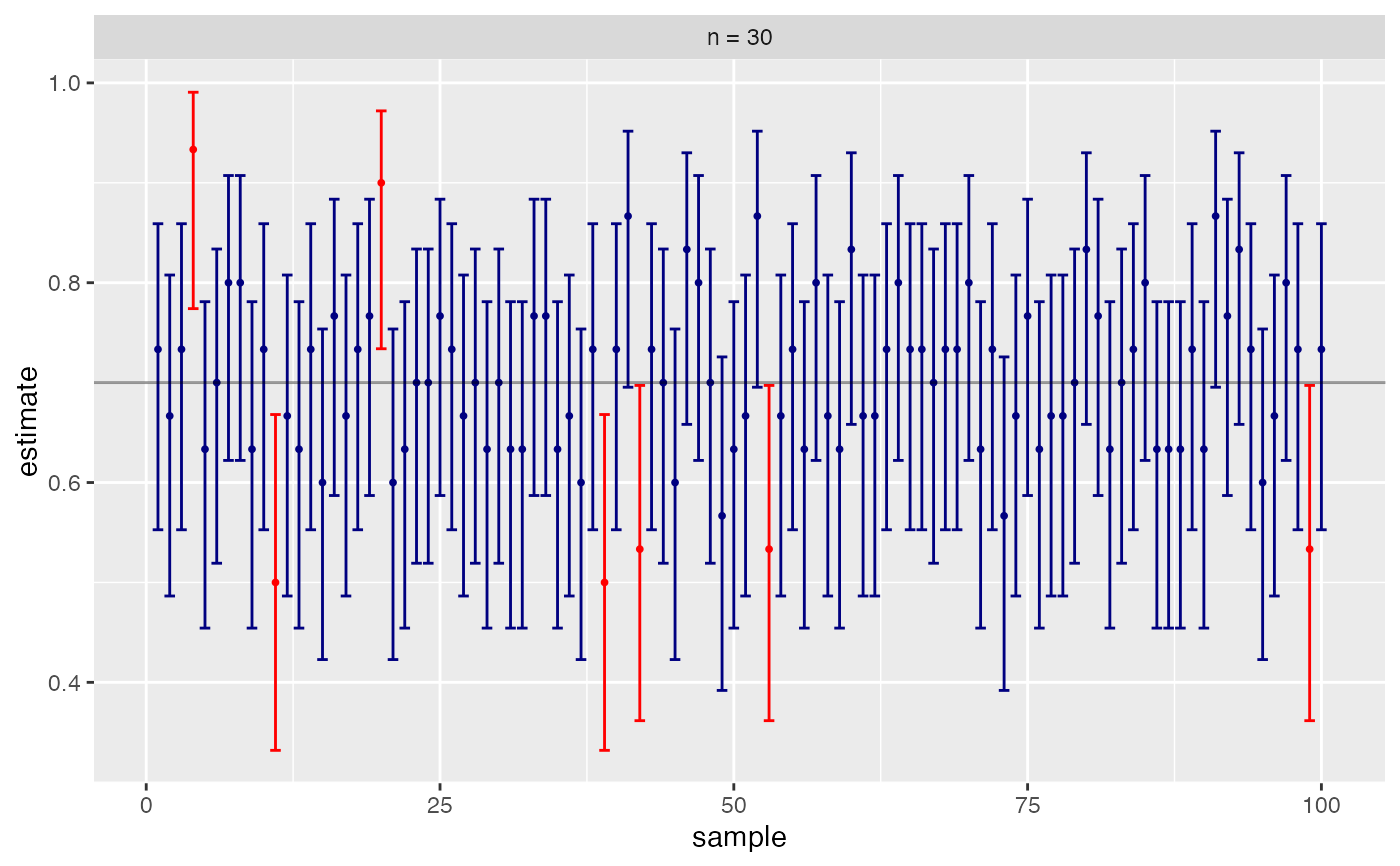This function automates the calculation of coverage rates for exploring the robustness of confidence interval methods.

CIsim(
n,
samples = 100,
rdist = rnorm,
args = list(),
plot = if (samples <= 200) "draw" else "none",
estimand = 0,
conf.level = 0.95,
method = t.test,
method.args = list(),
interval = function(x) {     do.call(method, c(list(x, conf.level = conf.level),
method.args))$conf.int }, estimate = function(x) { do.call(method, c(list(x, conf.level = conf.level), method.args))$estimate },
verbose = TRUE
)

## Arguments

n

size of each sample

samples

number of samples to simulate

rdist

function used to draw random samples

args

arguments required by rdist

plot

one of "print", "return", "horizontal", or "none" describing whether a plot should be printed, returned, printed with horizontal intervals, or not generated at all.

estimand

true value of the parameter being estimated

conf.level

confidence level for intervals

method

function used to compute intervals. Standard functions that produce an object of class htest can be used here.

method.args

arguments required by method

interval

a function that computes a confidence interval from data. Function should return a vector of length 2.

estimate

a function that computes an estimate from data

verbose

print summary to screen?

## Value

A data frame with variables lower, upper, estimate, cover ('Yes' or 'No'), and sample

is returned invisibly. See the examples for a way to use this to display the intervals graphically.

## Examples

# 1000 95% intervals using t.test; population is N(0,1)
CIsim(n=10, samples=1000)
#> Interval coverage:
#>     cover
#> n      Low   Yes  High
#>   10 0.038 0.944 0.018
# this time population is Exp(1); fewer samples, so we get a plot
CIsim(n=10, samples=100, rdist=rexp, estimand=1)
#> Interval coverage:
#>     cover
#> n     Low  Yes High
#>   10 0.07 0.92 0.01# Binomial treats 1 like success, 0 like failure
CIsim(n=30, samples=100, rdist=rbinom, args=list(size=1, prob=.7),
estimand = .7, method = binom.test, method.args=list(ci = "Plus4"))
#> Interval coverage:
#>     cover
#> n     Low  Yes High
#>   30 0.02 0.98 0.00